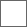• 13回复贴，共1

### 光子螺旋子粒子的折射n=c/u
n=1/sinγ，其中，γ为全反射临界角。

u=c/n=csinγ，其中，n光速折叠率，对应光子折射率。

u=c/n
n为介质中折叠率，等于折射率。即
n=c/u=1/sinγn=c/u

u=L0/t 其中，实际直线距离只有L0，而对应光程为L，c=L/t，所以，
u=cL0/L=c/n

n=L/L0n=c/u=λ0ν0/λν

n=c/u=λ0/λ

b=λ0
c=Nb=ν0λ0
c=Nb=L/t=L0n/t=nu

n=f(n0,N,Ne)

n0是介质固有属性，Ne是介质固有绕转转速，只有与光子转速N，三者协同，才能确定对应的折射率。#### 扫二维码下载贴吧客户端